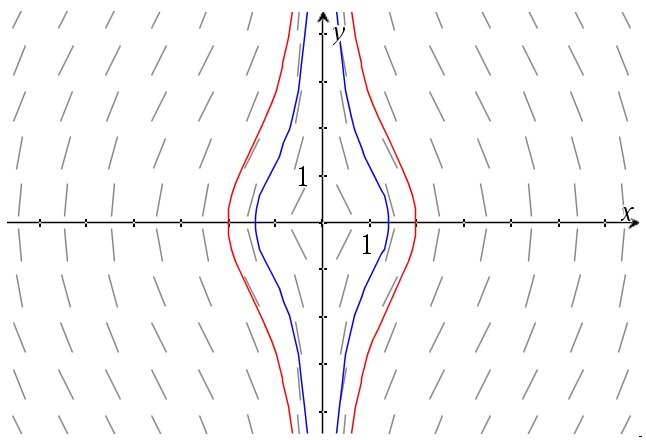# Homogenous differential equations – a first type

Up a level : Differential Equations
Previous page : Homogenous functions
Next page : Linear differential equationsSay we have an equation of the form$\frac{{dy}}{{dx}} = f\left( {\frac{y}{x}} \right)$

This we will solve by doing a variable substitution. Let$y = vx$

That means that$\frac{{dy}}{{dx}} = \frac{{d(vx)}}{{dx}} = \frac{{dv}}{{dx}}x + v\frac{{dx}}{{dx}} = \frac{{dv}}{{dx}}x + v$

This gives us that our original equation will become$\frac{{dv}}{{dx}}x + v = f\left( v \right)$

so$\frac{{dv}}{{dx}}x = f\left( v \right) - v$

and$\frac{{dv}}{{f\left( v \right) - v}} = \frac{{dx}}{x}$

Now we can integrate both sides separately. In practice it will often end up way easier than what it looks like from the above.

Example 1

We have already solved equations of this form, but let us try to solve one of them using this method to see that we get the same result. Let us look at$\frac{{dy}}{{dx}} = - \frac{x}{y}$

The substitutions above gives us$\frac{{dv}}{{dx}}x + v = - \frac{x}{{vx}} = - \frac{1}{v}$

This gives us$\frac{{dv}}{{dx}}x = - \frac{1}{v} - v$

or$\frac{{dv}}{{ - \frac{1}{v} - v}} = - \frac{{vdv}}{{1 + {v^2}}} = \frac{{dx}}{x}$

We can now do the substitution$\left| {\begin{array}{*{20}{c}} {w = 1 + {v^2}} \\ {dw = 2vdv} \\ {\frac{{dw}}{2} = vdv} \end{array}} \right.$

to get$- \frac{{dw}}{{2w}} = \frac{{dx}}{x}$

or, after integrations$- \frac{1}{2}\ln |w| = \ln |x| + {C_1}$

or$\ln |w| = - 2\ln |x| + {C_2}$

and thus$w = C{x^{ - 2}}$

or$w = C{x^{ - 2}}$

or , given that y=vx$1 + \frac{{{y^2}}}{{{x^2}}} = C{x^{ - 2}}$

Then we multiply through by x2 to get${x^2} + {y^2} = C$

I.e. the same solution as before, but in a way harder way. So let us look at an example where this method actually helps.

Example 2

Say we have$xyy' + {x^2} + {y^2} = 0$

We can rewrite this as$y' = - \frac{{{x^2} + {y^2}}}{{xy}} = - \frac{x}{y} - 1/\left( {\frac{x}{y}} \right)$

So it is actually an equation of the desired form.

Next we do the substitutions, and I suggest you do it on the original equation. We get$xvx\left( {\frac{{dv}}{{dx}}x + v} \right) + {x^2} + {(vx)^2} = 0$

or$v{x^2}\left( {\frac{{dv}}{{dx}}x + v} \right) + {x^2} + {v^2}{x^2} = 0$

or,${x^2}\left( {v\left( {\frac{{dv}}{{dx}}x + v} \right) + 1 + {v^2}} \right) = 0$

By the null factor law either x=0 or$v\left( {\frac{{dv}}{{dx}}x + v} \right) + 1 + {v^2} = 0$

This gives us$\begin{gathered} \frac{{dv}}{{dx}}x = \frac{{ - 1 - {v^2}}}{v} - v = \frac{{ - 1 - {v^2}}}{v} - \frac{{{v^2}}}{v} \hfill \\ \quad \quad = \frac{{ - 1 - 2{v^2}}}{v} \hfill \\ \end{gathered}$

or$- \frac{v}{{1 + 2{v^2}}}dv = \frac{{dx}}{x}$

The LHS we can solve by the substitution$\left| {\begin{array}{*{20}{c}} {w = 1 + 2{v^2}} \\ {dw = 4vdv} \\ {\frac{{dw}}{4} = vdv} \end{array}} \right.$

to get$- \frac{{dw}}{{4w}} = \frac{{dx}}{x}$

Integration gives us$- \frac{1}{4}\ln |w| = \ln |x| + {C_1}$

or$\ln |w| = - 4\ln |x| + {C_2}$

and$\ln |w| = \ln |{x^{ - 4}}| + {C_2}$

This gives us$w = {C_3}{x^{ - 4}}$

and$1 + 2{v^2} = C{x^{ - 4}}$

or, given that y=vx$1 + 2{\left( {\frac{y}{x}} \right)^2} = C{x^{ - 4}}$

or$1 + 2{\left( {\frac{y}{x}} \right)^2} = 1 + 2\frac{{{y^2}}}{{{x^2}}} = C{x^{ - 4}}$

Multiplying through by x4 gives us${x^4} + 2{x^2}{y^2} = C$

A graph of this is shown below.Interestingly enough the slope field is scale invariant (as you could figure out from how the equation is defined).

We will look at this example again later on.Up a level : Differential Equations
Previous page : Homogenous functions
Next page : Linear differential equationsLast modified: Mar 30, 2019 @ 16:18